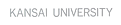### Engineering Science MajorMathematics

Cohomological Aspects in Mathematics

ProfessorKUSUDA Masaharu

Research Topics

① Crossed product of a Hilbert C*-module
② Properties of invariant states in a C*-dynamical system
③ Crossed product of a C*-algebra
④ Fixed point algebra of a C*-algebra by compact group actions
⑤ Mathematical physics, quantum statistical Mechanics

Key Words

Functional Analysis, Operator Algebras, C*-algebras, C*-Dynamical System, C*-Crossed Product, Extension of States, Hereditary C*-Subalgebra, Hilbert C*-Module, Morita Equivalence, Scattered C*-Algebra

Cohomological Aspects in Mathematics

ProfessorTAKEGOSHI Kensho

Cohomological Aspects in Mathematics

ProfessorFUJIOKA Atsushi

website

afujiokaResearch Topics

① Differential geometry related to integrable systems
② Geometric variational problems
③ Affine differential geometry

Key Words

Harmonic maps, Minimal surfaces, Surfaces with constant mean curvature, Integrable systems, Variational problems, Affine differential geometry

Cohomological Aspects in Mathematics

ProfessorMURABAYASHI Naoki

murabayaResearch Topics

① The arithmetic of abelian varieties with complex or quaternionic multiplication
② The relationship between abelian varieties and automorphic forms

Key Words

Abelian Variety, Complex Multiplication, Quaternionic Multiplication, Field of Moduli, Field of Definition, Defining Equation, Rational Point

Applications

Crytography Theory

Cohomological Aspects in Mathematics

ProfessorYANAGAWA Kohji

yanagawaResearch Topics

① Combinatorial commutative algebra
② Application of the derived category and sheaf theory to the above area
③ Oriented Matroid

Key Words

Stanley-Reisner Ring, Derived Category, Constructible Sheaf, Local Duality, Dualizing Complex, Poicare- Verdier Duality, (Affine)Oriented Matroid

Applications

Computational Algebra

Cohomological Aspects in Mathematics

Associate ProfessorWAKUI Michihisa

Research Topics

① Quantum invariants of knots and 3-manifolds
② Representation categories of Hopf algebras

Key Words

Topology, Tensor Category, Representation, Topological Field Theory, Hopf Algebra, Quantum Group, Knot, 3-Manifold, Subfactor, Triangulation

Applications

Natural Science

Probability and Statistics

ProfessorUEMURA Toshihiro

website

t-uemuraResearch Topics

① Global path properties for Markov Processes
② Regularity problem for Dirichlet forms
③ Construction of Feller semi-groups in terms of integro-differential operators

Key Words

Symmetric Stable-like Processes, Jump-diffusion, Dirichlet Forms, Martingale Additive Functional, Recurrence, Transience, Regularities, Ergodicity

Applications

Estimates for Stationarity of Markov Chains, Determination of Option Prices in the Discrete Model Case, Foundations of Probabilistic Risk Analysis

Probability and Statistics

ProfessorTAKEDA Masayoshi

Research Topics

① Stochastic calculus of symmetric Markov processes
② Spectral properties of symmetric Markov operators
③ Large deviations for additive functionals
④ Quasi-stationary distribution, penalization problem
⑤ Criticality theory for Schrödinger operators

Key Words

Symmetric Markov process, Additive functional, Large deviation, Dirichlet form

Probability and Statistics

ProfessorNAGAI Hideo

nagaihResearch Topics

① Large deviation control problems
② Risk-sensitive control and utility maximization
③ Optimal investment and consumption

Key Words

Large deviation control, Risk-sensitive control, Utility maximization, Stochastic differential equations, H-J-B equations, Robustness, Uncertainty

Applications

Financial engineering, Mathematical finance

Probability and Statistics

Associate ProfessorYAMAZAKI Kazutoshi

website

Research Topics

① Levy processes
② Optimal stopping, Optimal control
③ Applications in finance and insurance

Key Words

Levy processes, reflected Levy processes, optimal stopping, optimal control, impulse control, stochastic differential equations, HJB equations

Applications

Mathematical finance, credit risk, insurance, queues, inventory management